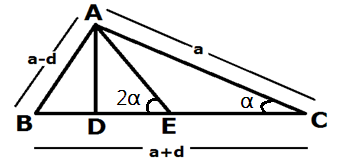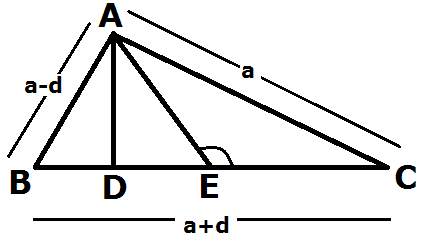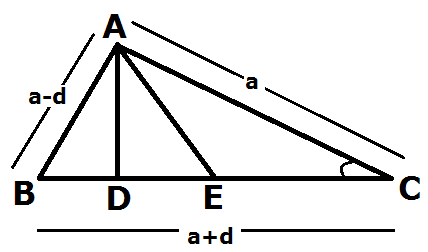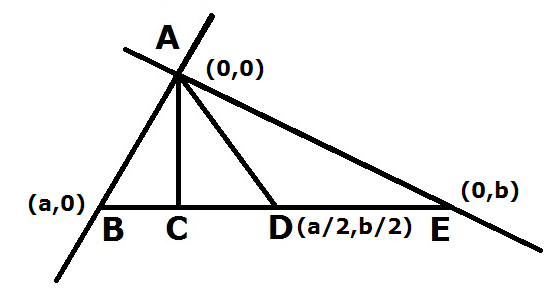Home » Articles » Four Proof of Theorem By PIYUSH GOEL

# Four Proof of Theorem By PIYUSH GOEL

Four Proof of Theorem (By PIYUSH GOEL)

Piyush Theorem: In a Right-Angled Triangle with sides in A.P

Theorem: In a Right-Angled Triangle with sides in A.P. Series, the distance between the point of intersection of median & altitude at the base is 1/10 Th the sum of other two sides.

This Theorem applies in Two Conditions:

1. The Triangle must be Right-Angled.

2. Its Sides are in A.P. Series.

1. Proof with TrigonometryDC Tan  α = DE Tan 2 α

(DE+EC)  Tan  α = DE Tan 2 α

DE Tan  α  + EC Tan  α = DE Tan 2 α

DE Tan  α  + EC Tan  α = 2 DE Tan  α / (1- Tan2  α   )

DE Tan  α   – DE Tan3  α + EC Tan  α –EC Tan3  α  = 2DE Tan  α

EC Tan  α –EC Tan3  α – DE Tan3  α = 2DE Tan  α – DE Tan  α

Tan  α (EC – EC Tan2  α – DE T an2  α )= DE Tan  α

DE Tan2  α  – DE = EC Tan2  α  – EC

-DE ( Tan2  α + 1) = -EC (1 – Tan2  α )

DE (sin2 α  /cos2 α + 1) = EC (1- sin2 α  /cos2 α )

DE (sin2 α + cos2 α /cos2 α ) = EC (cos2 α sin2 α /cos2 α )

DE (sin2 α  + cos2 α ) = EC(cos2 α  sin2 α )

DE (sin2 α  + cos2 α ) = EC (cos2 α  sin2 α )           where (sin2 α  + cos2 α =1) & (cos2 α  sin2 α = cos2 α  )              DE= EC cos2 α

cos α   =a/a+d   & sin α = (a-d)/ (a +d)

cos2 α  = a2/ (a +b) 2

sin2 α  = (a-d) 2/ (a+ d) 2

DE= EC (cos2 α    – sin2 α )

= EC (a2 / (a +b) 2 – (a-d) 2/ (a +d) 2

= EC (a2 – (a-d) 2/ (a +d) 2

= EC (a –a +d) (a+ a-d)/ (a+ d) 2

= EC (d) (2a -d)/ (a+ d) 2

= (a +d)/2(d) (2a -d)/ (a +d) 2 ————- where EC= (a +d)/2

= (d) (2a -d)/2(a +d)

= (d) (8d -d)/2(4d+d)       ——————where a= 4d (as per the Theorem)

= 7d2 /2(5d)

= 7d /10

= (3d+4d)/10= (AB+AC)/10

1. Proof with Obtuse Triangle TheoremAC2=EC2 +AE2 +2CE.DE       where EC = (  a +d) /2,AE=( a +d)/2

a2 = (a +d/2)2 + (a+ d/2)2 + 2(a +d)/2DE

= (a +d/2) (a+d+2DE)

= (a +d/2) (a+d+2DE)   where a=4d

16d2 = (5d/2) (5d+2DE)

32d/5 = 5d + 2DE

32d/5 – 5d = 2DE

32d -25d/5 = 2DE

DE =7d/10

= (3d+4d)/10 = (AB+AC)/10

1. Proof with Acute Triangle TheoremAB2= AC2+BC2 – 2BC.DC

(a-d) 2= a2 + (a+ d) 2 -2(a+ d) (DE+EC)         where AB= (a-d), AC=a, BC =( a +d) & EC= (a +d)/2

(a-d) 2 – (a +d)2 = a2  -2(a +d)(DE+EC)

(a- d –a-d) (a -d +a +d)  = a2 -2(a+ d) (2DE+a+d)/2

2(-2d) (2a) = 2a2 -2(a +d) (2DE+a+d)

-8ad 2a2 = -2(a +d) (2DE+a+d)

-2a (4d   +a) = -2(a +d) (2DE+a+d)

a (4d  + a) = (a +d)(2DE+a+d)

4d (4 d + 4d) = (4d+d) (2DE+4d+d)

4d (8d) = (5d) (2DE+5d)

32d2/5d =   (2DE+5d)

32d/5 =   (2DE+5d)

32d/5 – 5d =   2DE

(32d – 25d)/5 =   2 DE

DE = 7d/10

= (3d+4d)/10 = (AB+AC)/10

4. Proof with Co-ordinates GeometryEquation of BE

Y – 0 =b-0/0-a(X – a)

Y = -b/a(X) + b——————- (1)

M1 = -b/a

For perpendicular

M1M2= -1

So M2=a/b

Equation of AC

Y – 0 = a/b(X-0)

Y=a/b(X) —————— (2)

Put Y value in equation (1)

a/b(X) + b/a(X) =b

X (a2+b2/a b) = b

X = ab2/ (a2 + b2)

To get Value of Y, put X value in equation (2)

Y = a/b (ab2/ (a2+b2)

Y = a2b/ (a2+b2)

Here we got co-ordinates of Point C – ab2/ (a2 + b2), a2b/ (a2+b2) and co-ordinates of point d is (a/2, b/2) because d is midpoint.

As per the “Theorem” a=z-d, b=z, c = z+ d

(z +d) 2= (z-d) 2+z2 from here z=4d so a=3d and b=4d

Put value of a & b

ab2/ (a2 + b2), a2b/ (a2+b2) & (a/2, b/2)

ab2/ (a2 + b2) = 48d/25

a2b/ (a2+b2) = 36d/25

a/ 2=3d/2

b/ 2 =4d/2

CD2= (48d/25 -3d/2)2-(36d/25-4d/2)2

= (96d-75d/50)2 + (72d-100d/50)2

= (21d/50)2 + (-28d/50)2

= (441d2/2500) + (784d2/2500)

= (1225d2/2500)

CD= 35d/50 = 7d/10

= 7d/10 = (3d+4d)/10 = (AB+AE)/10Posts by SpeakBindas Editorial Team.